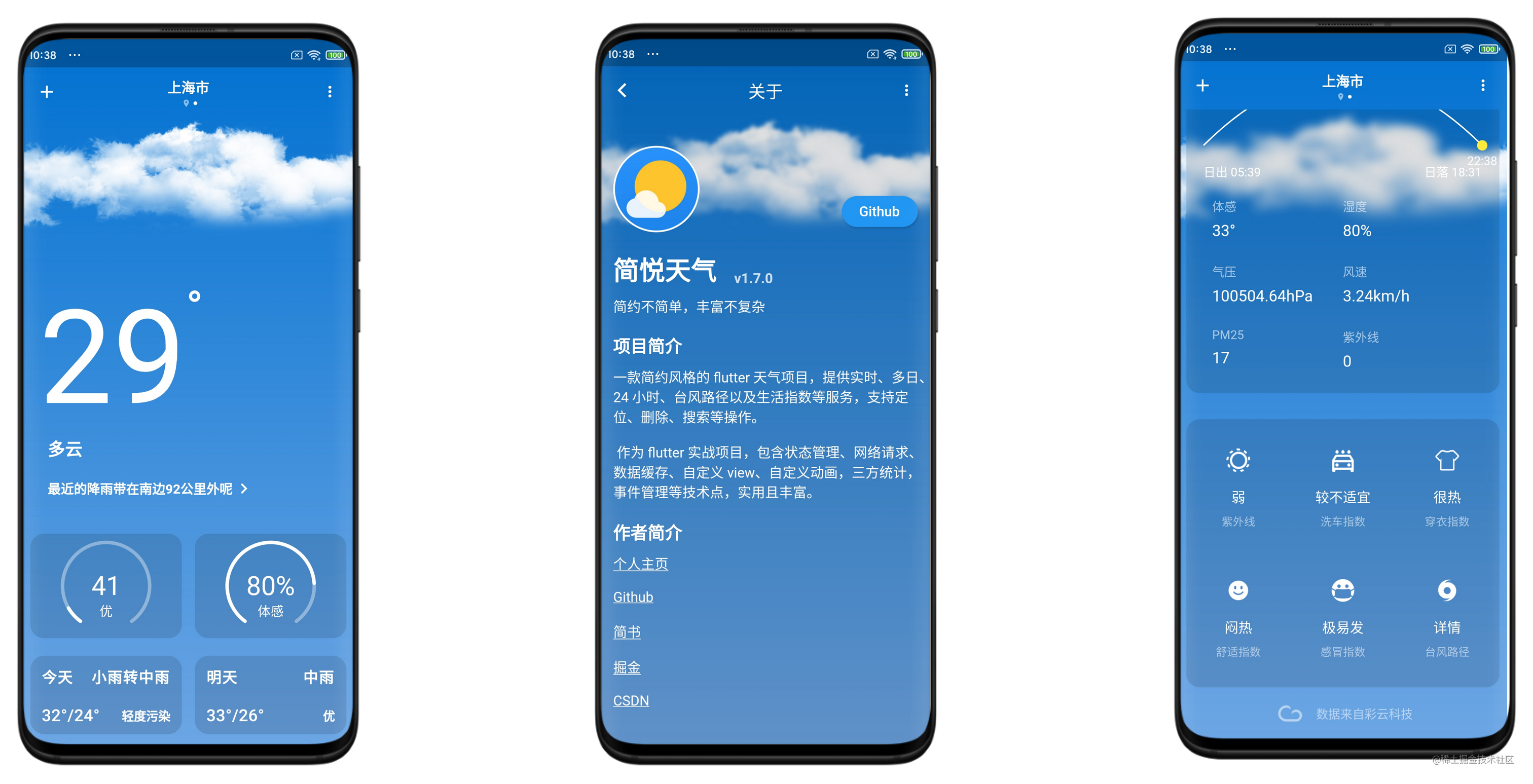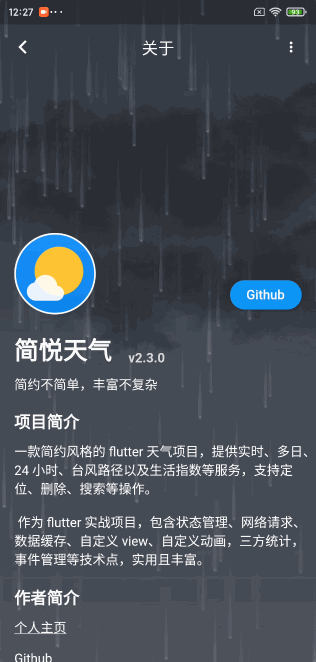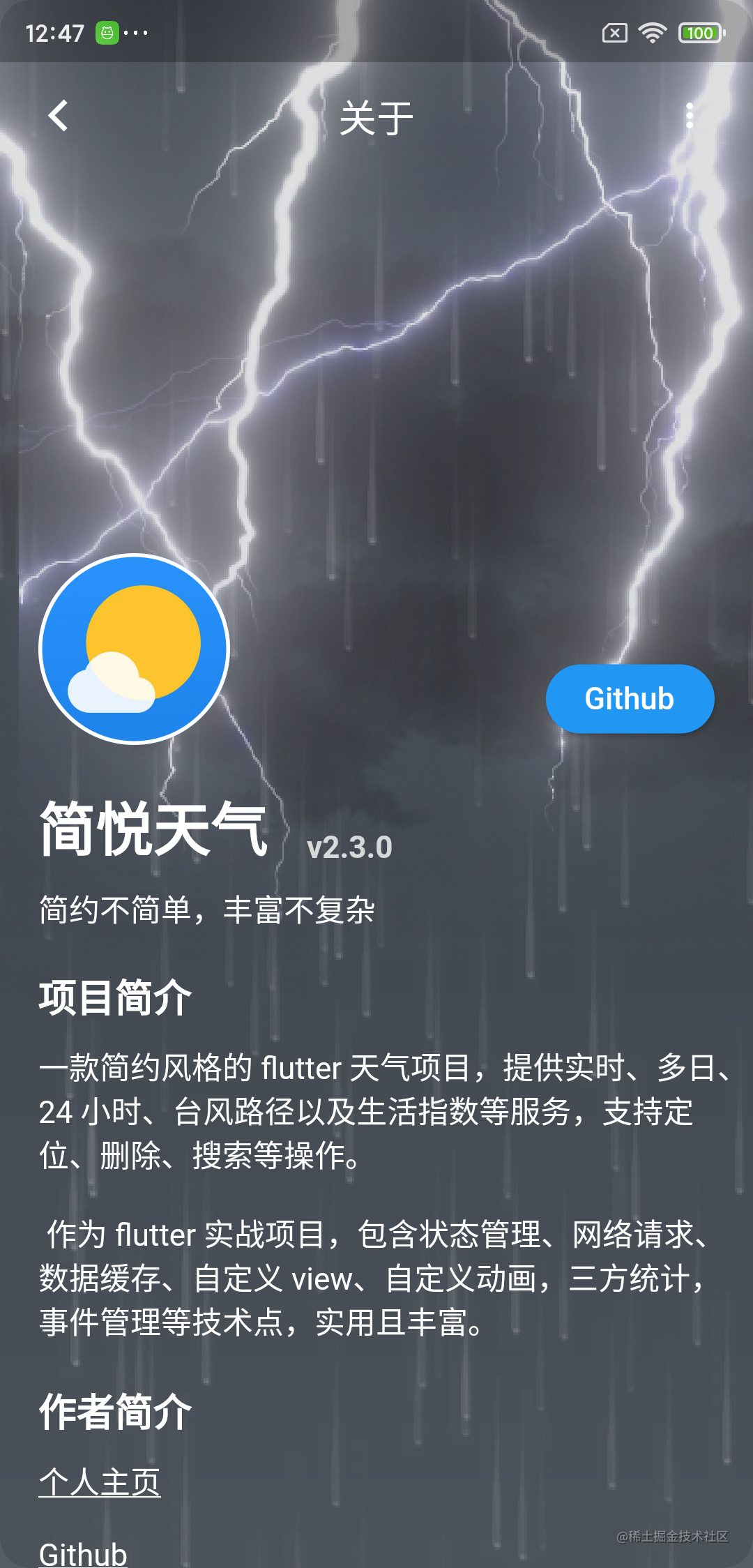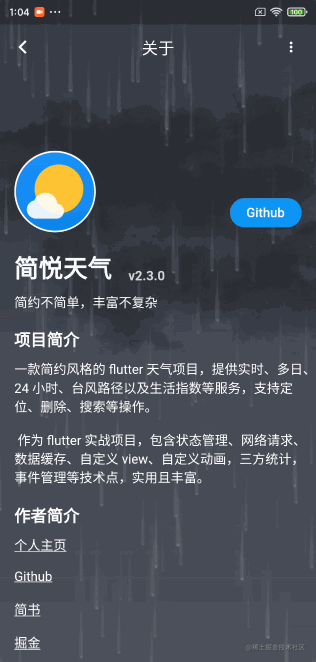# 『Flutter-绘制篇』实现炫酷的雷电特效

## 前言• 背景颜色层。从上到下的渐变效果
• 云层。只有一种图片，对其位移、数量、染色做不同变化达到不同效果
• 信息层。包括雨雪、雷暴和晴晚流星效果## 准备

``````  Future<void> fetchImages() async {
weatherPrint("开始获取雷暴图片");
var image1 = await ImageUtils.getImage('assets/images/lightning/lightning0.webp');
var image2 = await ImageUtils.getImage('assets/images/lightning/lightning1.webp');
var image3 = await ImageUtils.getImage('assets/images/lightning/lightning2.webp');
var image4 = await ImageUtils.getImage('assets/images/lightning/lightning3.webp');
var image5 = await ImageUtils.getImage('assets/images/lightning/lightning4.webp');
weatherPrint("获取雷暴图片成功： \${_images?.length}");
}

``````class ThunderParams {
ui.Image image;
double x;
double y;
double alpha;
int get imgWidth => image.width;
int get imgHeight => image.height;

ThunderParams(this.image);

void reset() {
x = Random().nextDouble() * 0.5.wp -  1 / 3 * imgWidth;
y = Random().nextDouble() * -0.05.hp;
alpha = 0;
}
}

`reset()` 方法用于在当前雷暴结束时，重新初始化参数信息。

## 绘制

``````  void drawThunder(ThunderParams params, Canvas canvas, Size size) {
if (params == null || params.image == null) {
return;
}
canvas.save();
var identity = ColorFilter.matrix(<double>[
1, 0, 0, 0, 0,
0, 1, 0, 0, 0,
0, 0, 1, 0, 0,
0, 0, 0, params.alpha, 0,
]);
_paint.colorFilter = identity;
canvas.drawImage(params.image, Offset(params.x, params.y), _paint);
canvas.restore();
}## 动画

TweenSequence 是一个动画序列，支持配置权重，以及对应的动画 Tween。这样，我们可以给 alpha 在 [0,1] 区间做动画时权重设置低一点，[1,0] 时权重高一点。

``````    var _animation = TweenSequence([
TweenSequenceItem(
tween: Tween(begin: 0.0, end: 1.0)
.chain(CurveTween(curve: Curves.easeIn)),
weight: 1),
TweenSequenceItem(
tween: Tween(begin: 1.0, end: 0.0)
.chain(CurveTween(curve: Curves.easeIn)),
weight: 3),
]);``````	_controller = AnimationController(duration: Duration(seconds: 1), vsync: this);
if (status == AnimationStatus.completed) {
_controller.reset();
Future.delayed(Duration(milliseconds: 10)).then((value) {
initThunderParams();
_controller.forward();
});
}
});

var _animation = TweenSequence([
TweenSequenceItem(
tween: Tween(begin: 0.0, end: 1.0)
.chain(CurveTween(curve: Curves.easeIn)),
weight: 1),
TweenSequenceItem(
tween: Tween(begin: 1.0, end: 0.0)
.chain(CurveTween(curve: Curves.easeIn)),
weight: 3),
]).animate(CurvedAnimation(
parent: _controller,
curve: Interval(
0.0, 1.0,
curve: Curves.ease,
),
));

``````Interval(
0.0, 0.3,
curve: Curves.ease,
)

Android